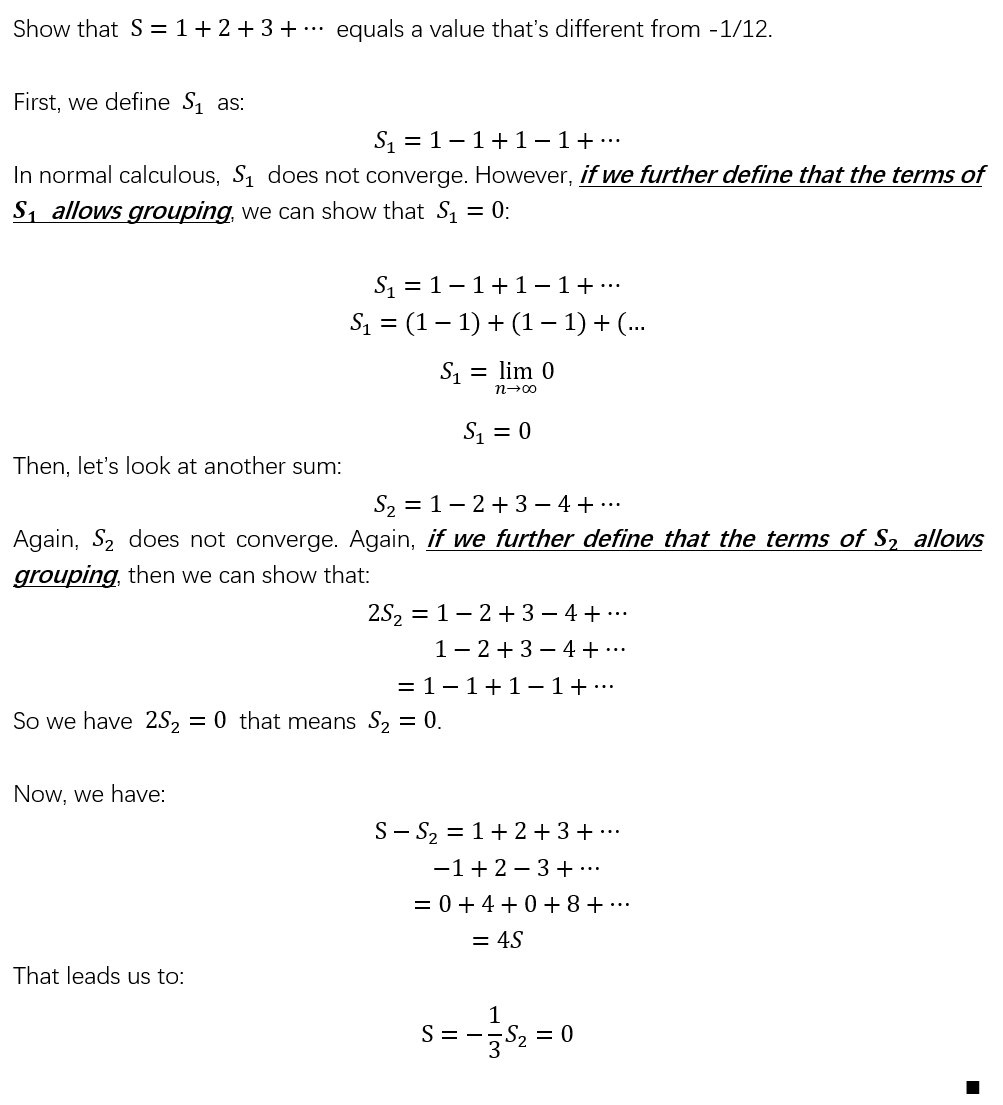# The sum of all natural numbers…

The notion of 1+2+3+4+…=-1/12 has become so wide spread that quite a few of my friends actually feel convinced that it’s actually a true statement in general, even though it is very counter intuitive.

The point is, in order to evaluate an expression, that expression has to be well-defined.

Expressions like a+b is well defined in the sense that we know it is a binary operation, we know what are we supposed to do to get the result.

Expressions like a+b+c+… is not well defined, because there are multiple operators involved, and the order of evaluation is not clearly specified.

Take the sum of the alternating series as an example:

1-1+1-1+1-1+1……

If you are allowed to group it as

(1-1)+(1-1)+(1-1)+……

then you might come to the conclusion that it converges to 0. But then if you regroup it as

1-(1-1)-(1-1)-……

Then you come to the conclusion that it converges to 1. As every CS students knows, the order of evaluation matters. If you don’t specify the order of evaluation, your expression is not well-defined.

As for 1+2+3+4+…=-1/12, the popular proof rely on the evaluation of another alternating series, 1-2+3-4+……, which does (not surprisingly) not converge.

To show the flaw of the popular proof, I came up with a counter proof that shows equals to 0. Here you are: